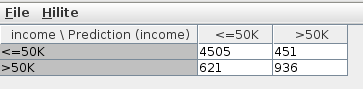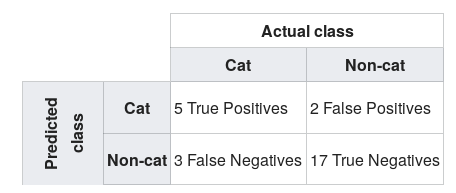# Score node, row and column say the same thing?

I am looking at the example “Building a simple classifier” under “Basic Examples”, and have a beginner question regarding the score node. I don’t get that both the columns and the rows say the same things, <=50k and >50k. What is the income and what is the prediction income?Income = actually observed,
Prediction income = predicted based on a model and train data

Ok, thanks I think I get that. But what I dont get is that the columns and the rows are called the same thing (<50K and >50K)? If either the rows or columns would say “income” and Prediction (income) that would make sense to me.
Please explainEdit: In wikipedia it says " Each row of the Matrix represents the instances in a predicted class while each column represents the instances in an actual class (or vice versa)."
The last vice versa…?

In wiki, they inverted the matrix.

The class labels are obviously the same for both actual and predicted.

Also notice the upper left corner “Income \ Predicted income”, meaning: actual values in the column and predicted values in the row.

Finally, if you click on View after right-clicking on the scorer node, you will have a nicer layout.

This is the source of confusion, in the wiki this is shown in a better way imho.That is what I did and what the screenshot is from, is it not supposed to look like that?